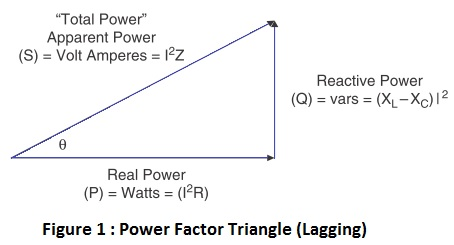# What is Power Factor and Why is it important?

### What is Power Factor?

In most electronic circuits it is essential to have the circuit work as a pure resistive load to the AC input line voltage. The degree by which the circuit comes nearer to a pure resistor is measured by the phase shift between the input voltage and input current and how well the waveform shape of the input current matches the waveform shape of the sinusoidal input voltage. The cosine of the phase angle between the input voltage and input current is defined as the Power Factor (See Figure 1). In other words we can define power factor as the ratio of the True (real) power (P) dissipated in the load to the apparent power (S) taken by the load, irrespective of the waveform.

### Power Factor Formula

Power Factor = True (Real) Power / Apparent PowerPower Factor Triangle (Lagging)
Where;
True (Real) Power = It is the time-averaged power, which results in heating or mechanical work being done, the rate of work being measured in true watts..

Reactive Power = It is the power required to produce the magnetic ﬁelds (lost power) to enable the real work to be done.

Apparent Power = It is considered as the total power that the power company supplies. This total power is the power supplied through the power mains to produce the required amount of real power. It is the time-averaged product of the RMS voltage and RMS current, measured at the input to the load, without adjustment for phase shift or distortion. Apparent Power formula is;

Apparent power (VA) = VRMS x IRMS

The power factor varies between 0 and 1, and normally can be either inductive (lagging, pointing up) or capacitive (leading, pointing down). When the current and voltage waveforms are in phase, the power factor is 1 [cos (0°) = 1] i.e. a PF of 1.0 (max.) equals to zero phase shift.  The entire need of making the power factor nearer to 1, is to make the power circuit look purely resistive (i.e. apparent power equal to real power).

In simple words; In the case of a purely resistive load, the voltage and current both are sinusoidal and in phase, and the true power is equal to the apparent power, giving the ideal power factor ratio of unity.

The previous definition of power factor associated to phase angle is valid when considering ideal sinusoidal waveforms for both current and voltage; however, most power supplies draw a non-sinusoidal current. If the current is not sinusoidal and voltage is sinusoidal, the PF has two factors;

1) Displacement factor related to phase angle
2) Distortion factor related to wave shape.

Below equation represents the relationship of the displacement and distortion factor as it belongs to power factor.

PF = [Irms (1)/ Irms] cosθ  =  Kd x Kθ

Where;
Irms (1) = Current’s fundamental component
Irms = Current’s RMS value

So, the need of the PFC circuit is to minimize the input current deformation or distortion and make the current in phase with the voltage. When power factor is not equal to 1, the current waveform does not trails the voltage waveform. This effect not only in power losses, but it may also cause harmonics that travel down the neutral line and disturb other devices connected to the line. The nearer the power factor is to 1, the nearer the current harmonics will be to zero as all the power is present in the fundamental frequency.

How fine the shape of the input current waveform matches the shape of the input voltage is determined by the total harmonic distortion (THD). A THD of 0% represents a pure sine wave (no distortion). This is the reason why we need a high PF and a low THD.

Why is it Important?

Now we will prove why power factor correction is needed or why is it important?

The following equation defines the amount of output power (Pout) available from a switchmode power supply:

Pout = Vo x Io x PF x Eff.

Where;
Pout = Output Wattage
Vo = Output Voltage
PF = Power Factor
Eff. = Efficiency

Below are two examples of power supplies with different power factors;

Consider: Vo = 120V
Io = 12A
Efficiency = 85%

• A switch-mode supply with 0.65 PF and 85% efficiency can only deliver (120 x 12 x 0.65 x 0.85) = 796 Watts (Pout).

• However, if the PF is improved to 0.98, the same power supply can now give output wattage as; (120 x 12 x 0.98 x 0.85) = 1200 Watts (Pout).

Second example shows increase in wattage and more availability of power from Power Supply. We can see reduction of losses from Power System.

Conclusion

With the above example we understood the Power Factor and Why is it needed. Also seen the need of Power Factor Correction. Hence Current and voltage both should be in phase to achieve good power factor and to avoid power loss.